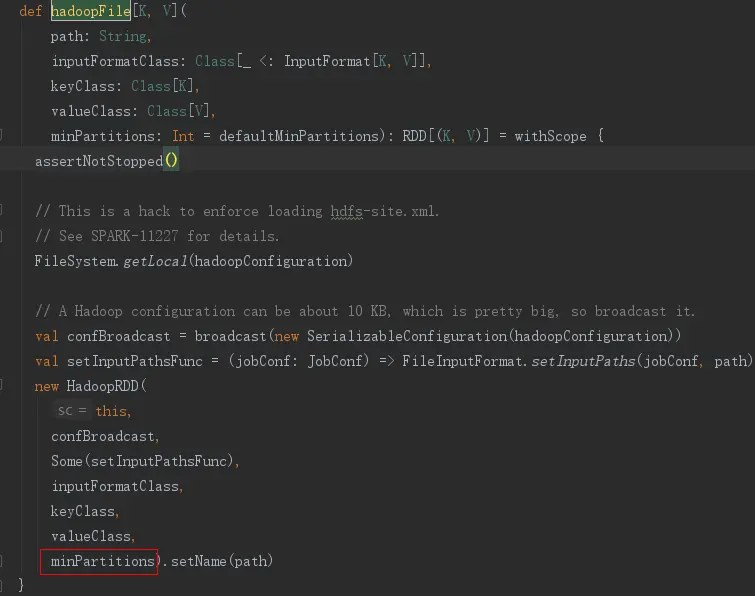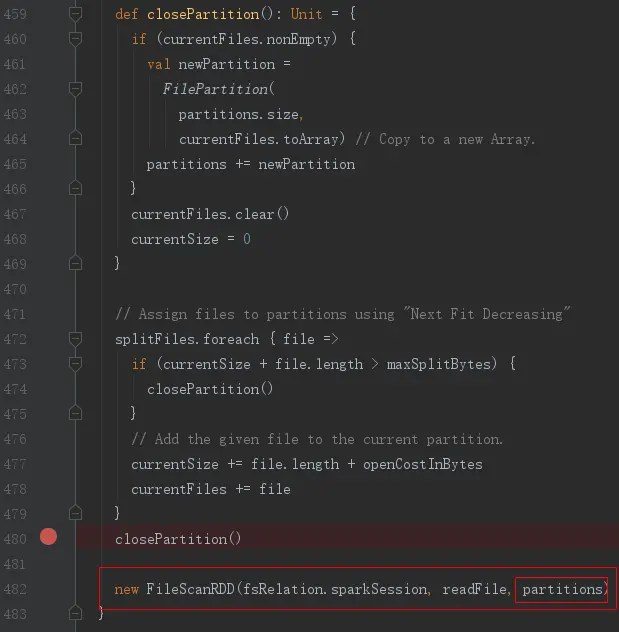# Spark source code analysis-textFile

Posted May 25, 20202 min read

## Overview

RDD is the core of Spark, and its creation method can be converted from other RDDs or created from a storage system, such as a local file system or hdfs. Among them, the textFile in SparkContext can produce RDD from the file system, its essence is that the RDD instance is newly created, and one of the important information is the partition. It will be described in detail below.

## textFile parsing

Call textFile can be used in the following way:

``````    SparkSession spark = SparkSession
.builder()
.appName("JavaWordCount")
.getOrCreate();
JavaRDD <String> lines = spark.sparkContext(). TextFile(args , 2) .toJavaRDD();``````

or:

``JavaRDD <String> lines = spark.read(). TextFile(args ). JavaRDD();``

Among them, calling the textFile in SparkContext will call the hadoopFile method in SparkContext.scala, the most critical is new HadoopRDD.There is a minPartitions in the parameters required by new HadoopRDD, which is passed in from the second parameter in the textFile function, such as 2 in the example.
The second way to call textFile will eventually call the createNonBucketedReadRDD method in DataSourceScanExec.scala, and finally the new FileScanRDD## Calculation of the number of partitions

The number of RDD partitions determines the number of tasks and needs attention. You can use the getNumPartitions method of the RDD to query the number of partitions of the RDD.
A minimum number of partitions can be passed in textFile minPartitions
The calculation logic in the source code is as follows:

``````long goalSize = totalSize/(long)(numSplits == 0? 1:numSplits); //totalSize is the total file size, numSplits is the minimum number of partitions passed in textFile minPartitions
long minSize = Math.max(job.getLong("mapreduce.input.fileinputformat.split.minsize", 1L), this.minSplitSize);
long blockSize = file.getBlockSize(); //Get the block size of hdfs, default 128M
long splitSize = Math.max(minSize, Math.min(goalSize, blockSize)); //splitSize is the size of 1 partition
long numPartitions = totalSize%splitSize == 0? totalSize/splitSize:totalSize/splitSize + 1;``````

Source tracking: